Unit 4: Estimating, Time, and Shapes

# Circle

The circle is a shape we all know.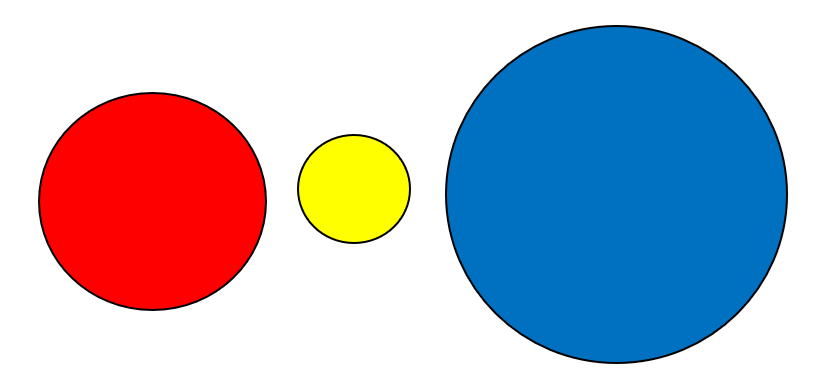These objects suggest the idea of a circle:

• rim of coffee cups and glasses
• top of lamp shades top of cans of food
• compact discs
• the ends of pipes and hoses (called the cross-section)
• the coloured part of your eye (the iris)

# Triangle

A triangle is a three-sided shape. Triangles have three sides and three angles.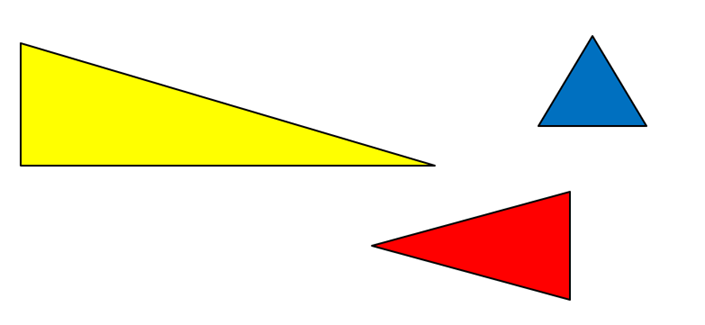Draw some different sized triangles here.

# Rectangle

A rectangle is a four-sided shape. Rectangles have four sides and four right angles (square corners).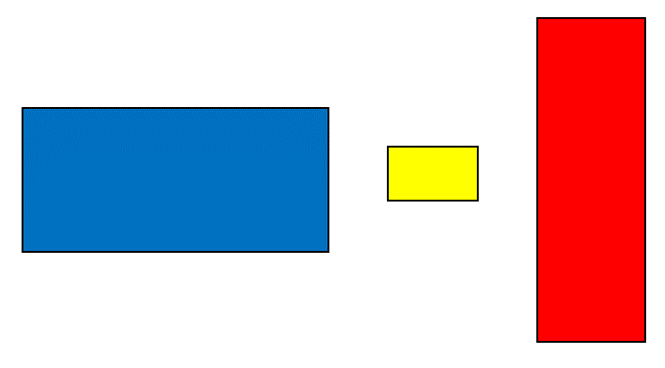Can you think of anything that has a rectangle shape? Write it here.

# Square

A square is a special kind of rectangle. Squares have square corners and all four sides are the same length.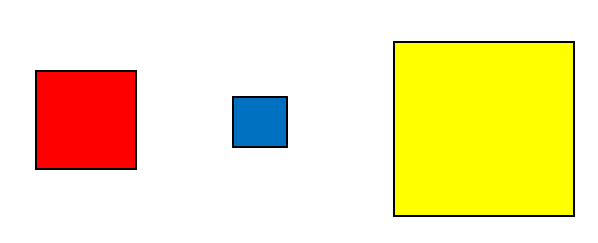Can you think of anything that has a square shape? Write it here.

Exercise 1

The following things give the idea of a shape. Write the name of the shape in each blank. Then draw the shape.

Example:

A cookie is a circle.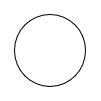1. A door is a          .
3. A yield sign is a          .
4. A room is usually a          .
5. A coin is a          .
6. A ten dollar bill is a          .
7. The rim of a jar is a          .
8. This warning sign is a          .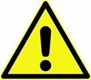9. A pizza is a          .

1. rectangle
2. rectangle
3. triangle
4. rectangle
5. circle
6. rectangle
7. circle
8. triangle
9. circle

Exercise 2

Look around the room and find each of the following shapes. Write the name on the line. Have your instructor check your answers.

Example:

A rectangle door.

1. A circle          .
2. A rectangle          .
3. A square          .
4. A triangle          .

Exercise 3

1. A rectangle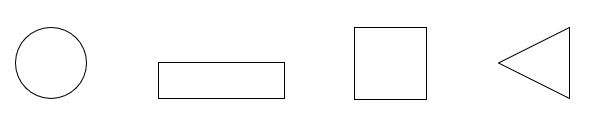2. A circle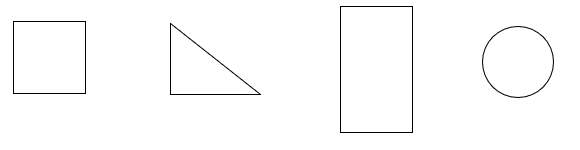3. A square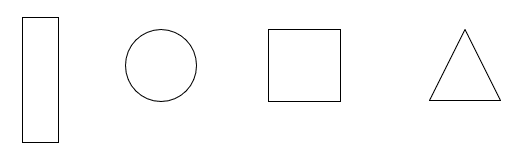4. A triangle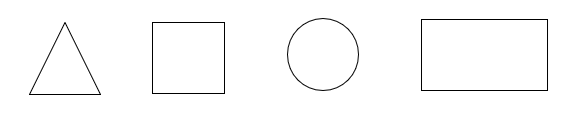Exercise 4

What shape are the following things? Write triangle, square, rectangle or circle.

1.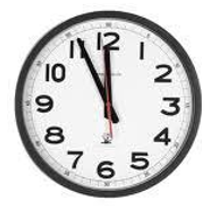2.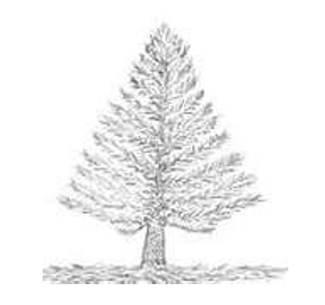3.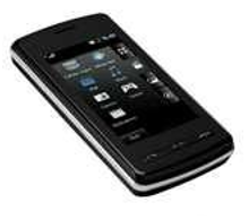4.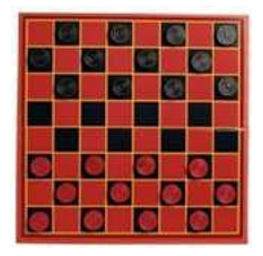5.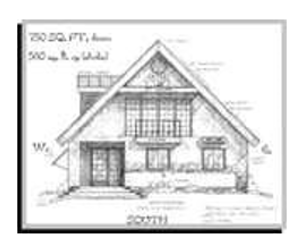6.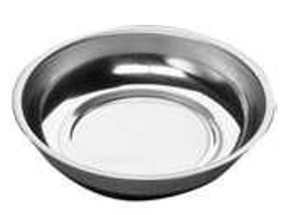7.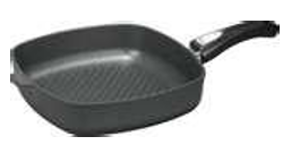8.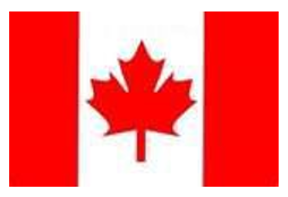1. circle
2. triangle
3. rectangle
4. square
5. rectangle or triangle
6. circle
7. square
8. rectangle